# A Rubik’s Cube puzzle relevant for post-quantum cryptography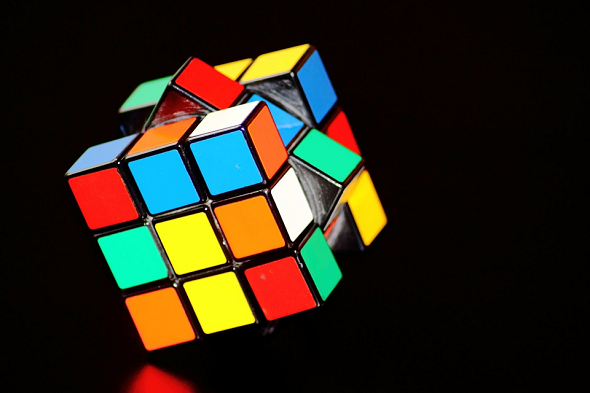A Rubik’s Cube can be used to define a crypto system that cannot be broken with quantum computers. Here’s a puzzle that shows the concept this system is built on. Can you solve it?

In September I will give a talk at the hacker conference 44CON in London (check here for my presentations calendar). It’s my second appearance at this conference. Last year …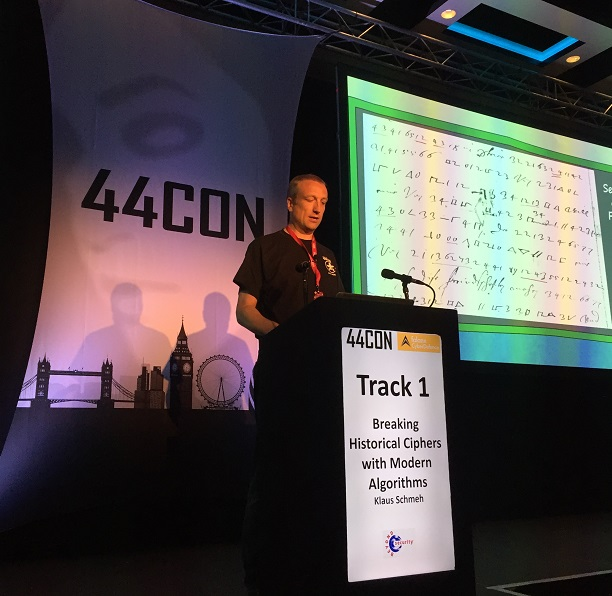… I spoke about Breaking Historical Ciphers with Modern Algorithms, intoducing many cases I covered on this blog.

At this year’s edition of 44CON I will talk about something completely different. The title of my presentation is How to Explain Post-Quantum Cryptography to a Middle School Student.

In fact, explaining post-quantum crypto algorithms to non-mathematicians is quite a challenge. Most of these methods involve sophisticated mathematics. In addition, the literature available is written for mathematicians, not for people only interested in understanding the basic concepts. The purpose of my presentation is to close this gap.

Among the crypto systems I will cover in my talk are the so-called non-commutative systems. I will explain them with a Rubik’s Cube. In the following, I will introduce a Rubik’s Cube puzzle that illustrates the mathematical problem this crypto system is built upon.

### Rubik’s Cube move sequences

First, we need to define Rubik’s Cube move sequences. Here is an example (we call it A):The following picture shows another move sequence (we call it B):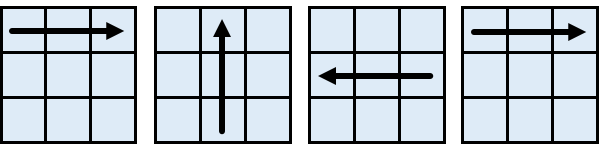A move sequence can have any length (including zero). The following move sequence has length five (the circle-shaped arrows denote a 90 degree turn; “f” stands for the front tier, “m” for the middle tier, “r” for the rear tier):Move sequences can be added. If A and B are defined as above, the following move sequence represents A+B: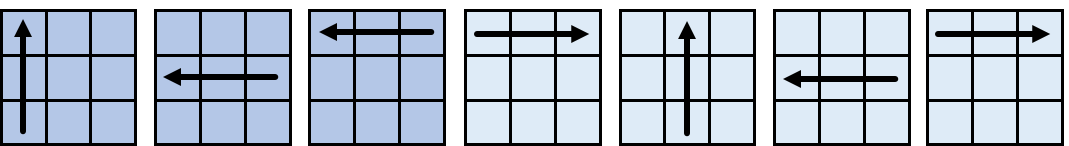As can be seen, the third and the fourth move of move sequence A+B neutralize each other. We can therefore omit these two moves. This means that A+B can also be written as follows: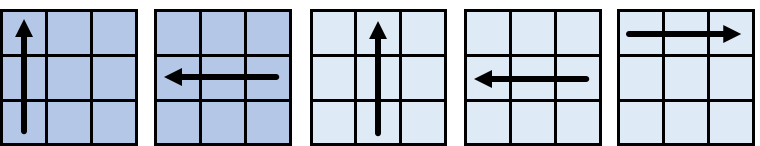It is clear A+B is not necessarily the same as B+A. This means that Rubik’s Cube move sequence addition is not commutative (unlike the addition of numbers).

Note that every move sequence has an inverse. If we add a move sequence to its inverse (or the other way round) we get the zero move sequence (i.e., the cube us not changed). The inverse of A is denoted -A. If this is A, …… here is -A: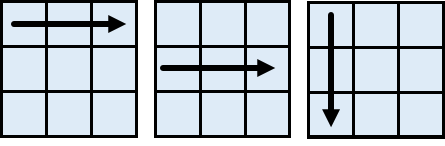It is clear that the move sequence A+(-A), which can also be written as A-A, does not change the cube. We therefore write A-A=0.

### A puzzle

Here’s a puzzle:

We have a move sequence A …… and a move sequence B:Find the move sequence X, for which X+A-X = B.

Here’s a diagram illustrating this puzzle:To make notation a little easier, we can write U for an upwards arrow, D for a downwards arrow, R for an arrow pointing to the right, and L for an arrow pointing to the left. The circle-shaped arrows are denoted as CW (clockwise) and CC (counter-clockwise). In addition we use the numbers 1, 2 and 3 (counted from left to right, top-down or front-to-rear). So, the move sequences in our puzzle can be noted as follows:

A = U1 L2 L1

B = R1 U2 L2 R1

If you can solve this puzzle try the following one, which involves longer move seqeunces:

A = CW2 R3 U2 L2 L1 R3 L1 D2

B = R1 U2 L2 CC3 L1 R2 CC2 U1

If you have found one of the solutions please leave a comment. And please tell us how you did it. I have to admit that I don’t know how to approach such a puzzle. It is clear, however, that a solution always exists.

### The conjgacy problem

To solve an equation of the kind X+A-X=B in a non-commutative group is referred to as the conjugacy problem. Finding a solution to an instance of the conjugacy problem can be quite difficult, if we deal with a large group. The conjugacy problem represents the revearsal of a one-way function, similar to computing the descrete logarithm or factorizing a prime number product.

It is possible to define a key exchange algorithm similar to Diffie-Hellman based on the conjugacy problem. Contrary to Diffie-Hellman, this algorithm is considered secure against quantum computer attacks.

It is out of scope to explain the non-commutative key exchange algorithm in this article. However, I will certainly cover it in my 44CON talk.

Further reading: How to Use a Rubik’s Cube for Encryption

## Kommentare (3)

1. #1 NorbertMediterranean
29. Juli 2018

It is clear, however, that a solution always exists.

Why is that clear? At first glance, I cannot see why there must be a solution X for any given A, B. Very interesting problem, though!

2. #2 Armin29. Juli 2018

@Klaus: Did you choose A and B to be conjugate? Because a solution X only exists if A and B are conjugate.

Counter example: let A=0, then B = X+A-X = X+0-X = X-X = 0. So you can’t choose B arbitrarily, B has to be also 0, which is the only conjugate of 0.

3. #3 Klaus Schmeh29. Juli 2018

@Armin: Sorry, you are right. The problem described is not solvable for all move sequences, but only for special ones. So, the puzzles are (probably) unsolvable.
I will rethink this issue and write a new blog post soon.